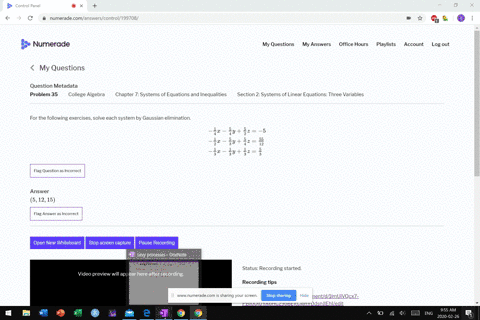Sign up for our free STEM online summer camps starting June 1st!View Summer Courses### For the following exercises, solve each system by…

04:37
MB

Need more help? Fill out this quick form to get professional live tutoring.

Get live tutoring
Problem 34

For the following exercises, solve each system by Gaussian elimination.
\begin{aligned}-\frac{1}{3} x-\frac{1}{8} y+\frac{1}{6} z &=-\frac{4}{3} \\-\frac{2}{3} x-\frac{7}{8} y+\frac{1}{3} z &=-\frac{23}{3} \\-\frac{1}{3} x-\frac{5}{8} y+\frac{5}{6} z &=0 \end{aligned}

$(40,-40,-40)$

## Discussion

You must be signed in to discuss.

## Video Transcript

a question. 34 is another system of equations to three unknowns. Um, and both in many fractions. Uh, think this time we're gonna try to do this with the fractions themselves. Will think that bull leaping, not clear the problem Fractions and see how that works to this one. I'm all right. So the first thing you want to dio is I will notice that both the first equation and the third equation both of these equations start out with negative 1/3 ex. Okay, so, um, so this and then so the same. So I could just attract them right now and some in But subtract these equations and I'm gonna subtract it. Going, Tom minus bottom. You could probably be the best. Okay, so top Marchioness. Right. So, um, negative five. Sorry. Negative. 18 minus negative. Five eights is gonna be four eights. Why so or eight Seems 1/2 so 1/2. Why? And then do it with the exit disease. They are getting Carmen on here. So 16 minus 56 is negative for six or name 2/3 z equals and then negative. 4/3 minus zero. All right, so I'm gonna combine the second and third equations. Um, problem here is that X is air, not the same. So I'm gonna, um, make them be the same cause I'm so moved by this equation through by two. So then I get negative 2/3 acts now the X's match and then, um, minus 10 force. Um, which is five. Why? And then 10 6 Jeez, plus 5/3 see peoples. And the two times zero is zero. So I combine, um, these two equations here. All right. And so 2/3 minus 2/3. So I get to Thursday. 2/3 0 Uh, negative. Five halves. Um, let me see. You didn't get common denominators. That's it. Ate up there so or or make this 20 eight. Wow. So we'll go down after all S o negative. 7/8 minus negative. 20 eights. ISS 13 eights, then 5/3 minus. Um, I'm going down. So 1/3 minus 5/3 is negative for thirds. See? And that equals negative. 23 3rd my zero. All right. And let me see. I wantto such a color here a little bit, so I want to track these. It looks like I can multiply this equation by two times, too. And I will get 4/3 for both sets of disease here. Okay, So top equation times too. Excuse me. Why, uh, minus 4/3 Z equals negativity. Thirds. Okay, um, now we've got used to equations, and I can subtract those. Um, so 13 eights minus eight is five eights. Why? And that's equal to these Cancels. An ominous attract here. So negative 23 minus negative. Eight is 15 3rd Um, negative 15 3rd So that's negative. Five. Multiply both sides, guys, times five. Okay, so I get why equals five cancels a white was negative. Eight. All right, now I want to find it's about ze Next. I got lots of equations disease, This one right here. So I used this equation. Changes color here for the Z. Um, so why is negative aids negative? Eight minus 4/3 z equals negative 8/3. Okay, um, add eight to both sides, So this be negative for Thurdsay? Um, a is 8 88 to this. So eight is 24 3rd So 24 3rd plus I'm negative. 8/3 is 16 3rd All right. Moved by both sides by three over negative four. It's all right. And I get Z equals. See the threes over this for Goes into 16 4 times the night before, Right? And then finally, I need to find X and something. Go back to any one of my first equations, and so what? I'm I She were this one right here. Zero only one thing, 1/3 offense. I get negative 1/3 acts minus, um, five seats. Times negative each plus five cents of, um, negative for equals zero. Um, so negative. 1/3 days for the H. Reduce your time's negatives. Positives of crossfire. And it will be minus minus 26. Last 26th is minus 13th zero. So that's what this is gonna be 15 3rd So these combined get 5/3 negative one eggs, um, equals negative. 5/3 most by both sides by three. Over negative one. And if x equals five. So the intersection here would you? Negative. And they work. All right,